Digits

How many five-digit numbers can be written from numbers 0.3,4, 5, 7 that is divided by 10 and if digits can be repeated.

Result

n =  500

Solution:Leave us a comment of example and its solution (i.e. if it is still somewhat unclear...):Be the first to comment!To solve this verbal math problem are needed these knowledge from mathematics:

See also our variations calculator. Would you like to compute count of combinations?

Next similar examples:

1. DigitsHow many natural numbers greater than 4000 which are formed from the numbers 0,1,3,7,9 with the figures not repeated, B) How many will the number of natural numbers less than 4000 and the numbers can be repeated?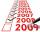Determine the number of integers from 1 to 106 with ending four digits 2006.
3. PIN - codesHow many five-digit PIN - code can we create using the even numbers?
4. Three-digitHow many three-digit natural numbers do not have the number 7?
5. Bridge cards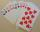How many bridge hands are possible containing 4 spades,6 diamonds, 1 club, and 2 hearts?
6. Toys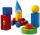3 children pulled 12 different toys from a box. Many ways can be divided toys so that each children had at least one toy?
7. Combinatorics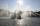The city has 7 fountains. Works only 6. How many options are there that can squirt ?
8. MedalsIn how many ways can be divided gold, silver and bronze medal among 21 contestant?
9. Olympics metalsIn how many ways can be win six athletes medal positions in the Olympics? Metal color matters.
10. Trainings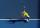The table contains tennis training schedule for Saturday's younger students during the winter indoor season. Before the start of the summer season is preparing a new training schedule. Tomas Kucera will be able to practice only in the morning, sisters Kova
11. A jackpot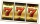How many times must I play this jackpot to win? A jackpot of seven games having (1 X 2), i. E. , home win or away win.
12. MetalsIn the Hockey World Cup play eight teams, determine how many ways can they win gold, silver and bronze medals.
13. Football league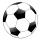In the 5th football league is 10 teams. How many ways can be filled first, second and third place?
14. Theorem proveWe want to prove the sentence: If the natural number n is divisible by six, then n is divisible by three. From what assumption we started?
15. Tricolors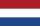From the colors - red, blue, green, black and white, create all possible tricolors.
16. VariationsDetermine the number of items when the count of variations of fourth class without repeating is 42 times larger than the count of variations of third class without repetition.
17. Six on diceWhat is the probability that when throwing two dice will fall at least one six?